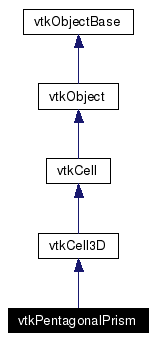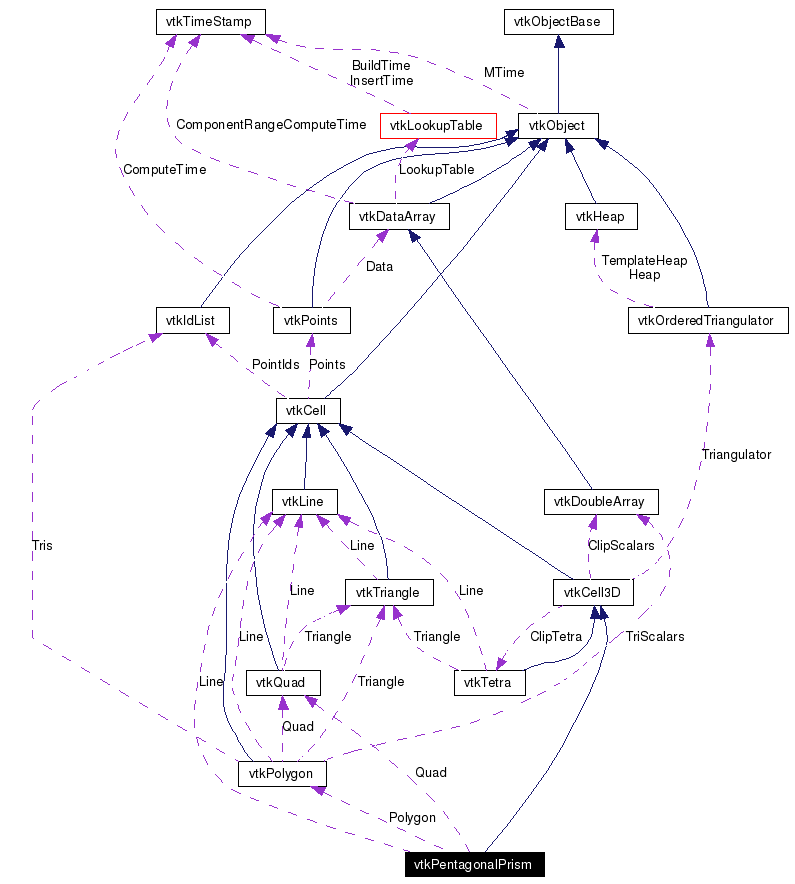# vtkPentagonalPrism Class Reference

`#include <vtkPentagonalPrism.h>`

Inheritance diagram for vtkPentagonalPrism:[legend]
Collaboration diagram for vtkPentagonalPrism:[legend]
List of all members.

## Detailed Description

a 3D cell that represents a prism with pentagonal base

vtkPentagonalPrism is a concrete implementation of vtkCell to represent a linear 3D prism with pentagonal base. Such prism is defined by the ten points (0-9) where (0,1,2,3,4) is the base of the prism which, using the right hand rule, forms a pentagon whose normal points is in the direction of the opposite face (5,6,7,8,9).

Thanks:
Thanks to Philippe Guerville who developed this class. Thanks to Charles Pignerol (CEA-DAM, France) who ported this class under VTK 4. Thanks to Jean Favre (CSCS, Switzerland) who contributed to integrate this class in VTK. Please address all comments to Jean Favre (jfavre at cscs.ch).
Tests:
vtkPentagonalPrism (Tests)

Definition at line 46 of file vtkPentagonalPrism.h.
int GetParametricCenter (double pcoords)
static void InterpolationFunctions (double pcoords, double weights)
static void InterpolationDerivs (double pcoords, double derivs)
static int * GetEdgeArray (int edgeId)
static int * GetFaceArray (int faceId)

## Public Types

typedef vtkCell3D Superclass

## Public Member Functions

virtual const char * GetClassName ()
virtual int IsA (const char *type)
int EvaluatePosition (double x, double *closestPoint, int &subId, double pcoords, double &dist2, double *weights)
void EvaluateLocation (int &subId, double pcoords, double x, double *weights)
int IntersectWithLine (double p1, double p2, double tol, double &t, double x, double pcoords, int &subId)
int Triangulate (int index, vtkIdList *ptIds, vtkPoints *pts)
void Derivatives (int subId, double pcoords, double *values, int dim, double *derivs)
double * GetParametricCoords ()
void JacobianInverse (double pcoords, double **inverse, double derivs)
void PrintSelf (ostream &os, vtkIndent indent)
virtual void GetEdgePoints (int edgeId, int *&pts)
virtual void GetFacePoints (int faceId, int *&pts)
int GetCellType ()
int GetCellDimension ()
int GetNumberOfEdges ()
int GetNumberOfFaces ()
vtkCellGetEdge (int edgeId)
vtkCellGetFace (int faceId)
int CellBoundary (int subId, double pcoords, vtkIdList *pts)

## Static Public Member Functions

static vtkPentagonalPrismNew ()
static int IsTypeOf (const char *type)
static vtkPentagonalPrismSafeDownCast (vtkObject *o)

## Protected Member Functions

vtkPentagonalPrism ()
~vtkPentagonalPrism ()

## Protected Attributes

vtkLineLine
vtkPolygonPolygon

## Member Typedef Documentation

 Reimplemented from vtkCell3D. Definition at line 50 of file vtkPentagonalPrism.h.

## Constructor & Destructor Documentation

 vtkPentagonalPrism::vtkPentagonalPrism ( ) ` [protected]`

 vtkPentagonalPrism::~vtkPentagonalPrism ( ) ` [protected]`

## Member Function Documentation

 static vtkPentagonalPrism* vtkPentagonalPrism::New ( ) ` [static]`
 Create an object with Debug turned off, modified time initialized to zero, and reference counting on. Reimplemented from vtkObject.

 virtual const char* vtkPentagonalPrism::GetClassName ( ) ` [virtual]`
 Reimplemented from vtkCell3D.

 static int vtkPentagonalPrism::IsTypeOf ( const char * type ) ` [static]`
 Return 1 if this class type is the same type of (or a subclass of) the named class. Returns 0 otherwise. This method works in combination with vtkTypeRevisionMacro found in vtkSetGet.h. Reimplemented from vtkCell3D.

 virtual int vtkPentagonalPrism::IsA ( const char * type ) ` [virtual]`
 Return 1 if this class is the same type of (or a subclass of) the named class. Returns 0 otherwise. This method works in combination with vtkTypeRevisionMacro found in vtkSetGet.h. Reimplemented from vtkCell3D.

 static vtkPentagonalPrism* vtkPentagonalPrism::SafeDownCast ( vtkObject * o ) ` [static]`
 Reimplemented from vtkCell3D.

 void vtkPentagonalPrism::PrintSelf ( ostream & os, vtkIndent indent ) ` [virtual]`
 Methods invoked by print to print information about the object including superclasses. Typically not called by the user (use Print() instead) but used in the hierarchical print process to combine the output of several classes. Reimplemented from vtkCell3D.

 virtual void vtkPentagonalPrism::GetEdgePoints ( int edgeId, int *& pts ) ` [virtual]`
 See vtkCell3D API for description of these methods. Implements vtkCell3D.

 virtual void vtkPentagonalPrism::GetFacePoints ( int faceId, int *& pts ) ` [virtual]`
 Get the list of vertices that define a face. The list is terminated with a negative number. Note that the vertices are 0-offset; that is, they refer to the ids of the cell, not the point ids of the mesh that the cell belongs to. The faceId must range between 0<=faceIdGetNumberOfFaces(). Implements vtkCell3D.

 int vtkPentagonalPrism::GetCellType ( ) ` [inline, virtual]`
 See the vtkCell3D API for descriptions of these methods. Implements vtkCell. Definition at line 61 of file vtkPentagonalPrism.h. References VTK_PENTAGONAL_PRISM.

 int vtkPentagonalPrism::GetCellDimension ( ) ` [inline, virtual]`
 See the vtkCell3D API for descriptions of these methods. Reimplemented from vtkCell3D. Definition at line 62 of file vtkPentagonalPrism.h.

 int vtkPentagonalPrism::GetNumberOfEdges ( ) ` [inline, virtual]`
 See the vtkCell3D API for descriptions of these methods. Implements vtkCell. Definition at line 63 of file vtkPentagonalPrism.h.

 int vtkPentagonalPrism::GetNumberOfFaces ( ) ` [inline, virtual]`
 See the vtkCell3D API for descriptions of these methods. Implements vtkCell. Definition at line 64 of file vtkPentagonalPrism.h.

 vtkCell* vtkPentagonalPrism::GetEdge ( int edgeId ) ` [virtual]`
 See the vtkCell3D API for descriptions of these methods. Implements vtkCell.

 vtkCell* vtkPentagonalPrism::GetFace ( int faceId ) ` [virtual]`
 See the vtkCell3D API for descriptions of these methods. Implements vtkCell.

 int vtkPentagonalPrism::CellBoundary ( int subId, double pcoords, vtkIdList * pts ) ` [virtual]`
 See the vtkCell3D API for descriptions of these methods. Implements vtkCell.

 int vtkPentagonalPrism::EvaluatePosition ( double x, double * closestPoint, int & subId, double pcoords, double & dist2, double * weights ) ` [virtual]`
 Given a point x return inside(=1) or outside(=0) cell; evaluate parametric coordinates, sub-cell id (!=0 only if cell is composite), distance squared of point x to cell (in particular, the sub-cell indicated), closest point on cell to x (unless closestPoint is null, in which case, the closest point and dist2 are not found), and interpolation weights in cell. (The number of weights is equal to the number of points defining the cell). Note: on rare occasions a -1 is returned from the method. This means that numerical error has occurred and all data returned from this method should be ignored. Also, inside/outside is determine parametrically. That is, a point is inside if it satisfies parametric limits. This can cause problems for cells of topological dimension 2 or less, since a point in 3D can project onto the cell within parametric limits but be "far" from the cell. Thus the value dist2 may be checked to determine true in/out. Implements vtkCell.

 void vtkPentagonalPrism::EvaluateLocation ( int & subId, double pcoords, double x, double * weights ) ` [virtual]`
 Determine global coordinate (x) from subId and parametric coordinates. Also returns interpolation weights. (The number of weights is equal to the number of points in the cell.) Implements vtkCell.

 int vtkPentagonalPrism::IntersectWithLine ( double p1, double p2, double tol, double & t, double x, double pcoords, int & subId ) ` [virtual]`
 Intersect with a ray. Return parametric coordinates (both line and cell) and global intersection coordinates, given ray definition and tolerance. The method returns non-zero value if intersection occurs. Implements vtkCell.

 int vtkPentagonalPrism::Triangulate ( int index, vtkIdList * ptIds, vtkPoints * pts ) ` [virtual]`
 Generate simplices of proper dimension. If cell is 3D, tetrahedron are generated; if 2D triangles; if 1D lines; if 0D points. The form of the output is a sequence of points, each n+1 points (where n is topological cell dimension) defining a simplex. The index is a parameter that controls which triangulation to use (if more than one is possible). If numerical degeneracy encountered, 0 is returned, otherwise 1 is returned. This method does not insert new points: all the points that define the simplices are the points that define the cell. Implements vtkCell.

 void vtkPentagonalPrism::Derivatives ( int subId, double pcoords, double * values, int dim, double * derivs ) ` [virtual]`
 Compute derivatives given cell subId and parametric coordinates. The values array is a series of data value(s) at the cell points. There is a one-to-one correspondence between cell point and data value(s). Dim is the number of data values per cell point. Derivs are derivatives in the x-y-z coordinate directions for each data value. Thus, if computing derivatives for a scalar function in a hexahedron, dim=1, 8 values are supplied, and 3 deriv values are returned (i.e., derivatives in x-y-z directions). On the other hand, if computing derivatives of velocity (vx,vy,vz) dim=3, 24 values are supplied ((vx,vy,vz)1, (vx,vy,vz)2, ....()8), and 9 deriv values are returned ((d(vx)/dx),(d(vx)/dy),(d(vx)/dz), (d(vy)/dx),(d(vy)/dy), (d(vy)/dz), (d(vz)/dx),(d(vz)/dy),(d(vz)/dz)). Implements vtkCell.

 double* vtkPentagonalPrism::GetParametricCoords ( ) ` [virtual]`
 Return a contiguous array of parametric coordinates of the points defining this cell. In other words, (px,py,pz, px,py,pz, etc..) The coordinates are ordered consistent with the definition of the point ordering for the cell. This method returns a non-NULL pointer when the cell is a primary type (i.e., IsPrimaryCell() is true). Note that 3D parametric coordinates are returned no matter what the topological dimension of the cell. Reimplemented from vtkCell.

 int vtkPentagonalPrism::GetParametricCenter ( double pcoords ) ` [inline, virtual]`
 Return the center of the wedge in parametric coordinates. Reimplemented from vtkCell. Definition at line 112 of file vtkPentagonalPrism.h.

 static void vtkPentagonalPrism::InterpolationFunctions ( double pcoords, double weights ) ` [static]`
 Pentagonal prism specific

 static void vtkPentagonalPrism::InterpolationDerivs ( double pcoords, double derivs ) ` [static]`
 Return the center of the wedge in parametric coordinates.

 static int* vtkPentagonalPrism::GetEdgeArray ( int edgeId ) ` [static]`
 Return the center of the wedge in parametric coordinates.

 static int* vtkPentagonalPrism::GetFaceArray ( int faceId ) ` [static]`
 Return the center of the wedge in parametric coordinates.

 void vtkPentagonalPrism::JacobianInverse ( double pcoords, double ** inverse, double derivs )
 Given parametric coordinates compute inverse Jacobian transformation matrix. Returns 9 elements of 3x3 inverse Jacobian plus interpolation function derivatives.

## Member Data Documentation

 vtkLine* vtkPentagonalPrism::Line` [protected]`
 Definition at line 102 of file vtkPentagonalPrism.h.

 vtkQuad* vtkPentagonalPrism::Quad` [protected]`
 Definition at line 103 of file vtkPentagonalPrism.h.

 vtkPolygon* vtkPentagonalPrism::Polygon` [protected]`
 Definition at line 104 of file vtkPentagonalPrism.h.

The documentation for this class was generated from the following file:

Generated on Tue Jan 22 00:06:44 2008 for VTK by1.4.3-20050530